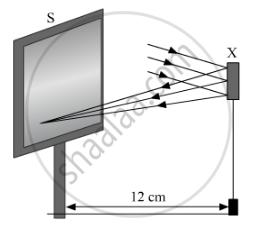# Study the Following Diagram and Select the Correct Statement About the Device 'X' : - Science

Study the following diagram and select the correct statement about the device 'X' :(A) Device 'X' is a concave mirror of radius of curvature 12 cm

(B) Device 'X' is a concave mirror of focal length 6 cm

(C) Device 'X' is a concave mirror of focal length 12 cm

(D) Device 'X' is a convex of mirror of focal length 12 cm

#### Solution

Because the light rays coming from infinity get reflected by the device 'X' and converge at a point at a distance 12 cm from it, the device 'X' is a concave mirror of focal length 12 cm.

Hence, the correct option is C.

Concept: Concave Mirror
Is there an error in this question or solution?
2014-2015 (March) Delhi Set 1

Share